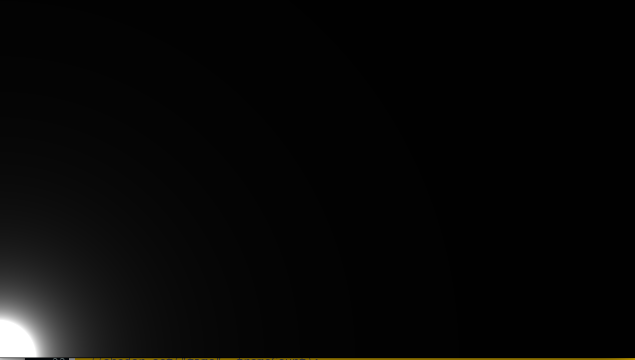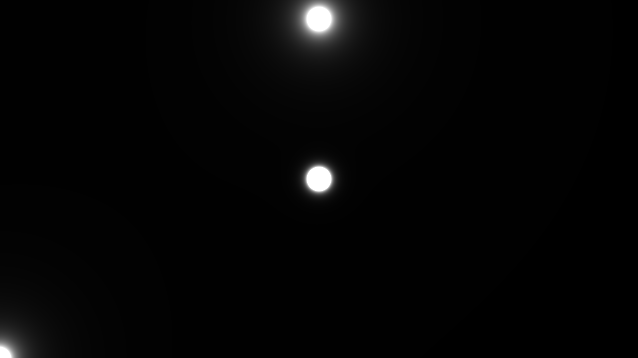#### Howdy, Stranger!

We are about to switch to a new forum software. Until then we have removed the registration on this forum.

#### In this Discussion

edited June 2016

I'm trying to get my head around glsl shaders. I am trying to get this shader from glslsandbox to work:

``````#ifdef GL_ES
precision mediump float;
#endif

#extension GL_OES_standard_derivatives : enable

uniform vec2 mouse;
uniform float time;
uniform vec2 resolution;

float w = resolution.x/2.0;
float h = resolution.y/2.0;
float PI = 3.141592653589793;

void main( void ) {
float move = w / 2.0;

vec2 pos1 = vec2(w + move * ( sin(time)), h);
vec2 pos2 = mouse * resolution;
vec2 pos3 = vec2( w + move * ( sin(time)),h + move * ( cos(time)));

float dist1 = length(gl_FragCoord.xy - pos1);
float dist2 = length(gl_FragCoord.xy - pos2);
float dist3 = length(gl_FragCoord.xy - pos3);

// 円のサイズ
float size = 25.0;

// distがsize以下であれば累乗根で潰す。
// この値が大きければsize以上離れている場所は0に近づくのでポワッとしなくなる
float color = 0.0;
color += pow(size / dist1, 5.0);
color += pow(size / dist2, 2.0);
color += pow(size / dist3, 2.0);

gl_FragColor = vec4(vec3(color / 1.0, color / 1.0, color / 1.0), 1.0);
}
``````

my processing code looks like this:

``````PShader shader;

void setup() {
size(640, 360,P3D);

}

void draw() {
background(0);

}
``````

It runs the program without any errors but all I get is a black background. What am I doing wrong, am I missing something in the code?

Thank you!

Tagged:

• do you need to pass `time` into the shader?

• it doesn't seem to make a difference.

I am drawing a rect over the canvas now which is resulting in this:but it's not moving nor following the mouse

``````PShader shader;

void setup() {
size(640, 360,P3D);

}

void draw() {
background(0);
rect(0, 0, width, height);
}
``````
• I've adjusted the code a little. All three ellipses show up now, two are moving. As soon as I move the mouse the one in the bottom corner disappears.``````PShader shader;

void setup() {
size(640, 360, P2D);

textureWrap(REPEAT);

}

void draw() {
rect(0,0,width,height);
//fill(255);
//ellipse(mouseX,mouseY,10,10);

})
``````

``````    float color1 = 0.0;
float color2 = 0.0;
float color3 = 0.0;
color1 += pow(size / dist1, 5.0);
color2 += pow(size / dist2, 2.0);
color3 += pow(size / dist3, 2.0);

gl_FragColor = vec4(vec3(color1 / 1.0, color2 / 1.0, color3 / 1.0), 1.0);
``````

and it's the green circle that goes away, so color2... now to work out why...

• ``````vec2 pos2 = mouse;
``````

is better. but you'll immediately notice a problem. fix it with

``````vec2 pos2 = vec2(mouse.x, resolution.y - mouse.y);
``````